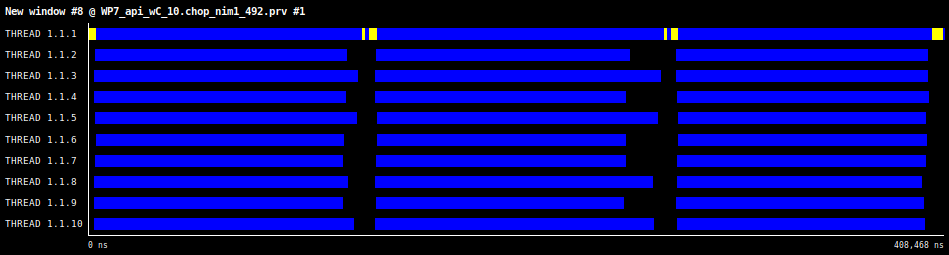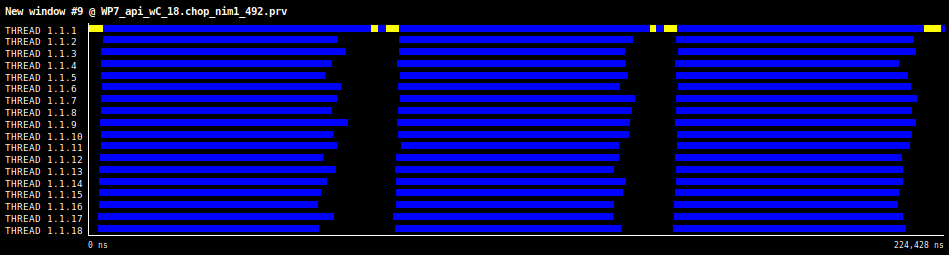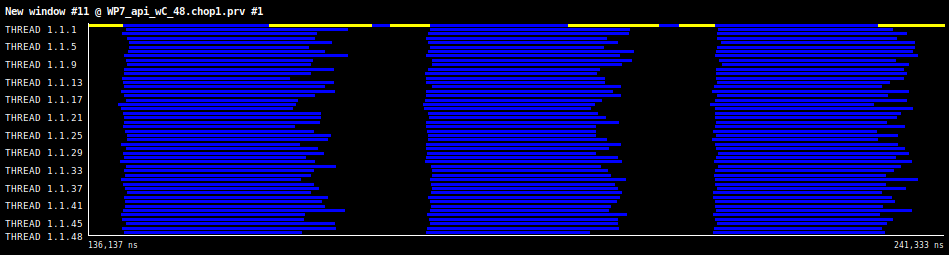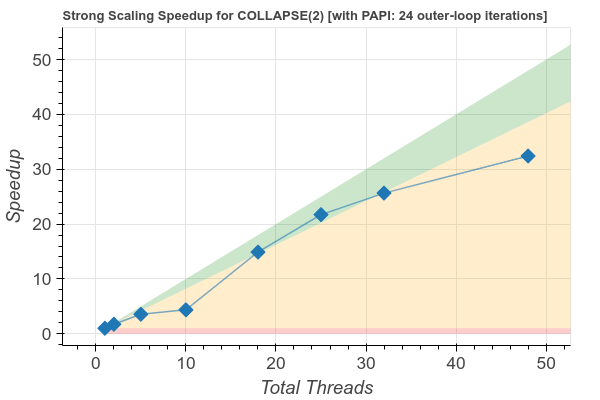# Collapsing OpenMP parallel loops

Pattern addressed: Lack of iterations on an OpenMP parallel loop

In some codes, the problem space is divided into a multi-dimensional grid on which the computations are performed. This pattern typically results in codes such as jCFD_Genesis, with multiple nested loops iterating over the grid, as shown in the following code snippet. (more...)

Required condition: OpenMP imbalance with nested loops.

In some codes, the problem space is divided into a multi-dimensional grid on which the computations are performed. This pattern typically results in codes with multiple nested loops iterating over the grid, as shown in the following code snippet.

DO K = 1, Nk
DO J = 1, Nj
DO  I = 1, Ni
!! work to do
END DO
END DO
END DO


In the loop above, the work is related to computations from a real-case, where the results of multiplication and addition between elements of multiple three-dimensional matrices are stored in an output three-dimensional matrix of the same size.

When the computations on the grid are independent one from another it is possible to compute the values in parallel. When implementing this pattern in using OpenMP it is common to parallelize the loops by using a regular !$OMP PARALLEL DO directive. However, poor load balance can occur because the number of iterations in the outer loop can’t be distributed evenly over the threads. One approach that may be implemented to solve the issue is to use the COLLAPSE(N) clause DO iter = 1, Niter !$OMP PARALLEL DO COLLAPSE(2) DEFAULT(NONE) SHARED(...)
DO K = 1, Nk
DO J = 1, Nj
DO  I = 1, Ni
!! work to do
END DO
END DO
END DO
!\$OMP END PARALLEL DO
END DO


The compiler will create a single loop by merging the outer N loops. In this way, the computation will run on a (linearized) space of size equal to the product of the size of the outer loops. The result is that the amount of work distributed to each thread may be better balanced, at the cost of doing the work of merging the loops.If using COLLAPSE(2) does not lead to good load balance, a COLLAPSE(3) clause may be tried.

There are specific conditions to be fulfilled to use the COLLAPSE clause (see, for more details on the conditions, here for an exhaustive list of rules). The most important ones are:

• The loops must form a rectangular iteration space;
• The loops must be perfectly nested;
• The associated do-loops must be structured blocks. Their execution must not be terminated by an EXIT statement.

In Figure 1, Extrae timeline windows for COLLAPSE(2) nested OpenMP loop with the two outer loops collapsed is shown for 10, 18 and 48 threads. As the number of threads increase very little imbalance is observed.Figure 1: Timeline view showing three outer iterations for a COLLAPSE(2) nested loop with Nk=22, Nj=42, Ni=82, and Niter=24.

Figure 2 shows the scaling plot for the collapsed nested loops in the code snippet above for Niter=24, Ni=492, Nk=22 and Nj=42.Figure 2: speedup plot for COLLAPSE(2) nested loop with Nk=22, Nj=42, Ni=82, and Niter=24.

In this case the K and J loops have been fused together by the compiler to create a space of dimension Nk*Nj which is larger wrt the maximum number of threads for this example (i.e.: 48). Only small imbalance is observed, the performance is improved with respect to the case without the COLLAPSE(2) directive, as the wall time decreases and the speedup for each run is always close to optimal (e.g.: >80%).

Recommended in program(s): OpenMP regular parallelization of nested loop ·

Implemented in program(s): OpenMP nested loop collapse ·